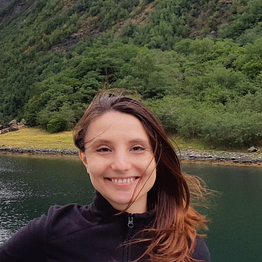# Subspace clustering

## Challenges in high dimensional spaces

1. What are the challenges of working with high dimensional data?
2. What is subspace clustering?
3. How to implement a subspace clustering algorithm in python
• it makes the visualization and thus understanding of the input difficult, it often requires applying a dimensionality reduction technique beforehand…

# Gentle introduction to Echo State Networks

## Also an intro to reservoir computing

1. What are Echo State Networks?
2. Why and when should you use an Echo State Network?
3. Simple implementation example in python

# Simple example of 2D density plots in python

## How to visualize joint distributions

• Use a Gaussian Kernel to estimate the PDF of 2 distributions
• Use Matplotlib to represent the PDF with labelled contour lines around density plots
• How to extract the contour lines
• How to plot in 3D the above Gaussian kernel
• How to use 2D histograms to plot the same PDF
`import numpy as npimport matplotlib.pyplot as pltimport scipy.stats as stfrom sklearn.datasets.samples_generator import make_blobsn_components = 3X, truth = make_blobs(n_samples=300, centers=n_components,                       cluster_std = [2, 1.5, 1],                       random_state=42)plt.scatter(X[:, 0], X[…`

# Adjacency matrix (A)## Madalina Ciortan

Computer science engineer, bioinformatician, researcher in data science